Status: Active

# MEASURING OCHRATOXIN A IN GRAPE JUICE, RECONSTITUTED GRAPE JUICE, CONCENTRATED GRAPE JUICE, AND GRAPE NECTAR, AFTER GOING THROUGH AN IMMUNOAFFINITY COLUMN AND HIGH LIQUID PERFORMANCE CHROMATOGRAPHY WITH FLUORESCENCE DETECTION

THE GENERAL ASSEMBLY,

IN VIEW of Article 2, paragraph iv of the Agreement of 3 April 2001 establishing the International Organisation of Vine and Wine,

AT THE PROPOSAL of the "Methods of Analysis" Sub-Commission,

# Measuring ochratoxin A in grape juice, reconstituted grape juice, concentrated grape juice, and nectar, after going through an immunoaffinity column and high liquid performance chromatography with fluorescence detection

Type IV method

For grape juice, reconstituted grape juice, and nectar: the application of the Method OIV-MA-AS315-10 of the Compendium of International Methods of Analysis of Wines and Musts.

For the concentrated grape juice: dilute the juices five times (m/m) with water, before initiate the item 5.1 of the Procedure. Consider this dilution in the final calculation of the OTA concentration (item 7), in the following equation: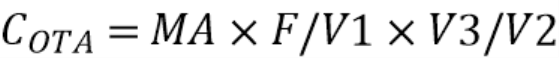in the value of the dilution factor (F).

Method proposal:

# Measuring ochratoxin A in grape juice, concentrated grape juice, reconstituted grape juice, and nectar after going through an immunoaffinity column and high liquid performance chromatography with fluorescence detection

Type IV method

## 1.Scope

This document describes the method used for determining ochratoxin A (OTA) in grape juice, reconstituted grape juice, concentrated grape juice, and nectar in concentrations ranging from 0.2 μg/L up to 10 μg/L.

## 2.Principle

This method use immunoaffinity column and high performance liquid chromatography (HPLC).

OTA is eluted with methanol and quantified by HPLC in reverse phase with fluorimetric detection.

## 3.Reagents and materials

### 3.1.Reagents for separation of the OTA on an immunoaffinity column. The reagents listed below are examples. Suppliers of immunoaffinity columns may offer dilution solutions and eluents suitable for their products. If so, it is preferable to use these products.

#### 3.1.5.Phosphate buffer (dilution solution).

Dissolve 60.0 g of Na2HPO4∙2H2O (3.1.1) and 8.8 g of NaH2PO4∙H2O (3.1.2) in 950 mL of water (3.1.4) and add more water to make up to 1 litre.

#### 3.1.6.Phosphate buffer saline (washing solution).

Dissolve 2.85 g of Na2HPO4∙2H2O (3.1.1), 0.55 g of NaH2PO4∙H2O (3.1.2) and 8.70 g of NaCl (3.1.3) in 950 mL of water (3.1.4) and add more water to make up to 1 litre.

### 3.2.Reagents for HPLC

#### 3.2.3.Mobile phase: water: acetonitrile: acetic acid, 99 : 99 : 2, v/v/v (approximate proportions.

Mix 990 ml of water (3.1.4) with 990 mL of acetonitrile (3.2.1) and 20 mL of acetic acid (3.2.2). In the presence of undissolved components, filter through a 0.45 μm filter. Degas (with helium, for example) unless the HPLC equipment used includes a degassing step.

### 3.3.Reagents for the preparation of the OTA stock solution

#### 3.3.3.Solvent mixture (toluene: acetic acid, 99 : 1, v/v)

Mix 99 parts in volume of toluene (3.3.1) with one part volume of acetic acid (3.2.2).

A commercial solution of standard concentration (around 50 μg/mL), with an analysis certificate stating the reference value and uncertainty of the concentration it is of preferential use than the use of OTA in solid form.

### 3.4.OTA stock solution

Dissolve 1 mg of OTA or the same content in a bulb, if the OTA was obtained in the form of film after evaporation, in the solvent mixture (3.3.3) to obtain a solution containing approximately 20 to 30 μg/mL of OTA.

To determine the exact concentration (if necessary), record the absorption spectrum between 300 and 370 nm in a quartz space with 1 cm of optical path while using the solvent mixture (3.3.3) as a blank. Identify maximum absorption and calculate the concentration of OTA (COTA) in μg/mL by using the following equation: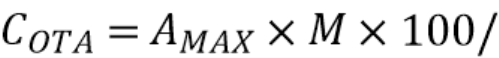ε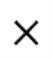δ

In which: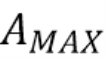= Absorption determined by the longest maximum wave (about 333 nm);

M = OTA molecular mass = 403.8 g/mol;

ε = OTA molar extinction coefficient in the solvent mixture (3.3.3) (ε = 544 m2/mol);

δ = optical pathway (cm).

This solution is stable at -18 °C for at least 4 years.

Standard OTA solution 2 μg/mL (toluene: acetic acid, 99 : 1, v/v)

Dilute the stock solution (3.4) with the solvent mixture (3.3.3) to obtain a standard solution of OTA with a concentration of 2 μg/mL.

This solution can be stored at 4 °C in a refrigerator. The stability should be tested in use.

## 4.Apparatus

Usual laboratory equipment and in particular, the following equipment:

### 4.9.Immunoaffinity columns specifically for OTA.

The column should have the total link capacity of at least 100 ng OTA. This will allow for a purification yield of at least 85 % when a diluted solution of the sample containing 100 ng OTA is passed through.

## 5.Preparations of samples (given as example)

### 5.1.For grape juice, reconstituted grape juice, and nectar

Pour 10 ml of the sample in a 100 mL conical flask. Add 10 mL of the dilution solution (3.1.5). Mix vigorously. Filter through glass microfibre filter (4.8). Filtration is necessary for cloudy solutions or when there is precipitation after dissolving.

### 5.2.For concentrated grape juice

First, dilute the juices five times (m/m) with water (3.1.4). Consider this dilution in the final calculation of the OTA concentration (item 8). Then, Pour 10 mL of the diluted sample in a 100 mL conical flask. Add 10 mL of the dilution solution (3.1.5). Mix vigorously. Filter through glass microfibre filter (4.8). Filtration is necessary for cloudy solutions or when there is precipitation after dissolving.

## 6.Procedure

### 6.1.Purification by immunoaffinity column (as an example)

Samples are diluted with a suitable solvent according to the instructions on the kit manufacturer. This solution is filtered and purified on an immunoaffinity column. Set up the immunoaffinity column (4.9) to the SPE system (4.6), and attach the reservoir (4.7)

Add 10 mL (equivalent to 5 mL of the sample) of the diluted solution in the reservoir. Put this solution through the immunoaffinity column at a flow of 1 drop per second. The immunoaffinity column should not become dry. Wash the immunoaffinity column with 5 mL of washing solution (3.1.6) and then with 5 ml of water (3.1.4) at a flow of 1 to 2 drops per second. Blow air through to dry column. Elute OTA in a glass tube (4.1) with 2 mL of methanol (3.1.7) at the rate of 1 drop per second. Evaporate the eluate to dryness at 50 °C with nitrogen. Dissolve again immediately in 250 μl of the HPLC mobile phase (3.2.3) and keep at 4 °C until the HPLC analysis.

### 6.2.HPLC analysis

Using the injection loop, inject 100 μl of reconstituted extract in the chromatography.

Operating conditions (as an example)

Flow: 1 mL/min.

Mobile phase: acetonitrile: water: acetic acid (99 : 99 : 2, v/v/v) (3.2.3)

Fluorescence detector: Excitation wavelength = 333 nm

Emitting wavelength = 460 nm

Volume of injection: 100 μL

## 7.Quantification of ochratoxin A (OTA)

The quantification of OTA should be calculated by measuring the area or the height of the peaks at the OTA retention time and compared to the calibration curve.

### 7.1.Calibration curve (as an example)

Prepare a calibration curve daily or every time chromatographical conditions change. Measure out 0.5 mL of the standard OTA solution (3.5) at 2 μg/mL in a glass tube (4.1) and evaporate the solvent using nitrogen. Dissolve again in 10 mL in the HPLC mobile phase (3.2.3) which was previously filtered using a 0.45 μm filter. This produces an OTA solution of 100 μg/L. Prepare at least 6 HPLC calibration solutions in five 5 mL calibrated volumetric flasks following Table 1 (as an example).

Complete each 5 mL standard solution with HPLC mobile phase (3.2.3).

Inject 100 μL of each solution in the HPLC.

Table 1. Calibration curve

 Calibration standards Std 1 Std 2 Std 3 Std 4 Std 5 Std 6 μL of mobile phase filtered HPLC (3.2.3) 4990 4975 4950 4900 4770 4500 μL of OTA solution at 100 μg/L 10 25 50 100 250 500 OTA concentration (μg/L) 0.2 0.5 1.0 2.0 5.0 10 Injected OTA (ng) 0.02 0.05 0.10 0.20 0.50 1.00

NOTES:

If the quantity of OTA in the samples is outside the calibration range, an appropriate dilution should occur. In these cases, the calculation of the final concentration (8) should be reviewed on a case by case basis.

# Check in the Guide to the critical points of the method of measuring ochratoxin A byimmunoaffinity column (appendix III- method OIV-MA-AS315-10).

## 1.Calculations

Calculate the quantity of OTA in the aliquot of the solution tested and injected in the HPLC column.

Calculate the concentration of OTA (COTA) in μg/L by using the following formula: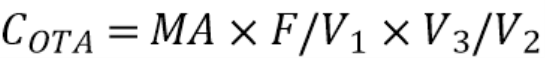Where:

MA is the mass of ochratoxin A (in μg) in the aliquot part of the template injected on the column and evaluated from the calibration curve.

F is the dilution factor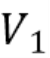is the sample volume to be analysed (0.01 L)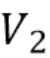the volume of the solution tested and injected in the column (100 μL)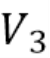is the volume of solution used to dissolve the dry eluate (250 μL)

The recovery rate of the immunoaffinity columns must be taken into consideration in the case of direct calibration by synthetic solutions.

## 2.Expression of the results

The amount of OTA is expressed in micrograms per liter (μg/L) with two significant figures taking into account uncertainty.

## 3.Characteristics of the method

An internal validation study was carried out for the purpose of assessing the suitability

of the method for grape juices, taking into account linearity, limits of detection and quantification and the accuracy of the method. The latter parameter was determined by defining the levels of precision and trueness of the method.

### 3.1.Linearity of the method

Based on the results obtained from the linear regression analysis, the method proved to be linear within the ranges shown in the Figure 1 and in the Table 2.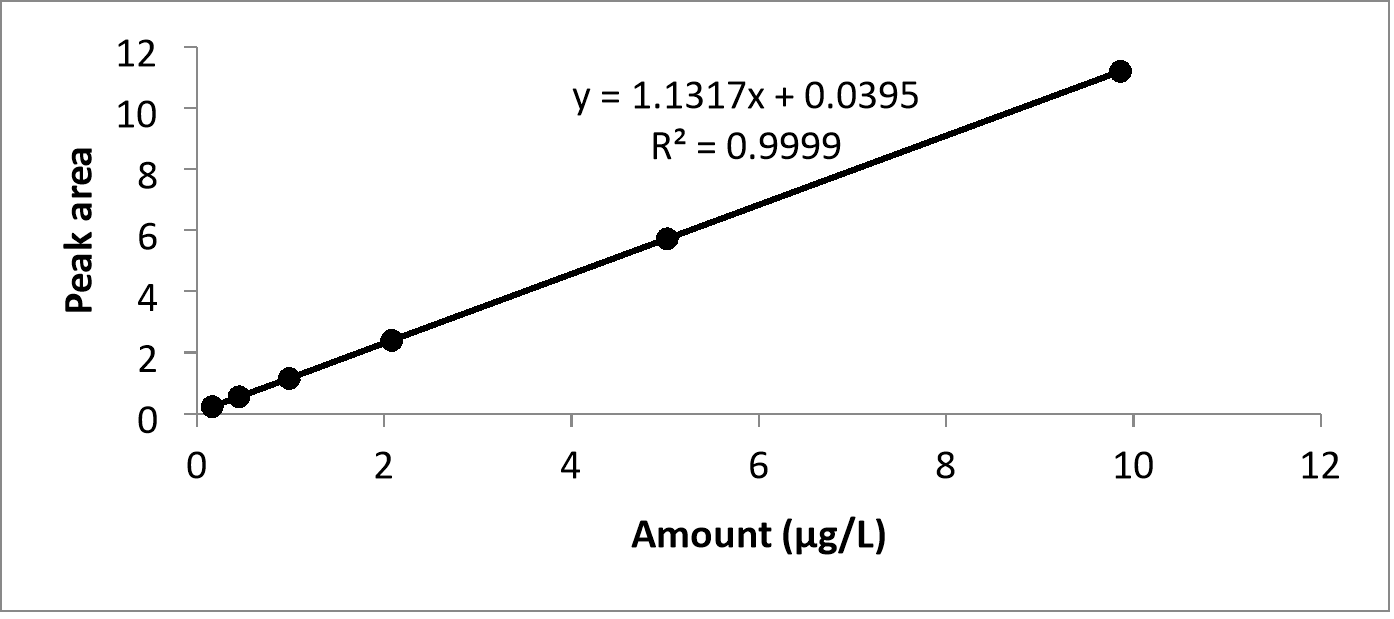Figure 1. OTA calibration curve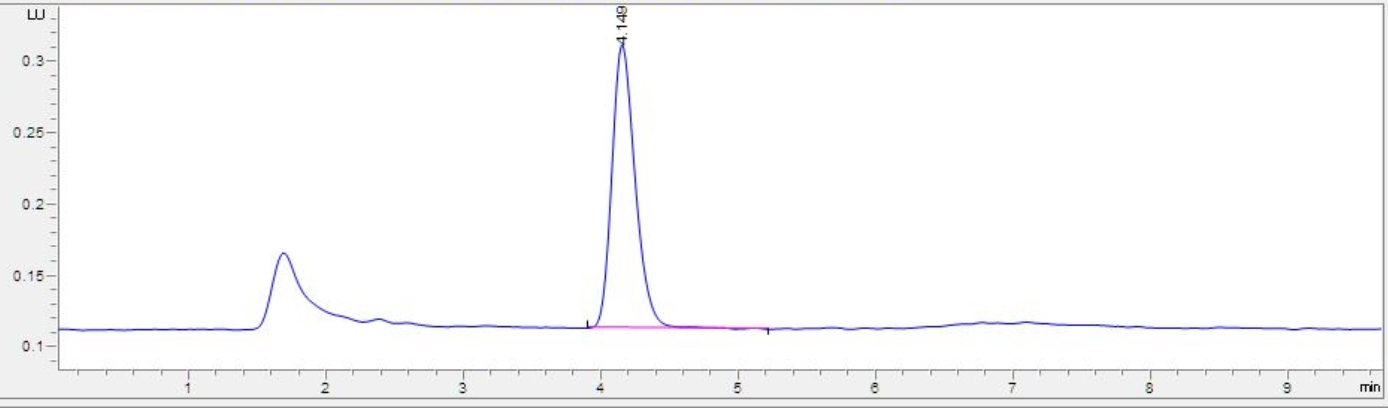Figure 2. Chromatogram of 2.0 μg/L OTA standard

### 3.2.Limit of detection and limit of quantification

The detection limit (LD) and the limit of quantification (LoQ) were calculated from a series of 8 analytical repetitions of a grape juice spiked with 0.10 μg/L of OTA and is equal to 3 standard deviations for the LD and 10 standard deviations for the LoQ (Table 2).

### 3.3.Precision of the method

The parameters taken into account were repeatability and reproducibility. Table 2 shows the values of these parameters. The repeatability was expressed as the relative standard deviation of measurements repeated in different concentrations found for grape juice. And the reproducibility was expressed as the average of the relative standard deviation of measurements of the same grape juice sample, made by different operators.

### 3.4.Trueness of the method

The percentage recovery was determined using a grape juice sample spiked in 6 concentrations levels of OTA, ranging from 0.10 μg/L to 10 μg/L. Each level was analyzed 5 times. An example of a recovery chromatogram is given in Figure 3.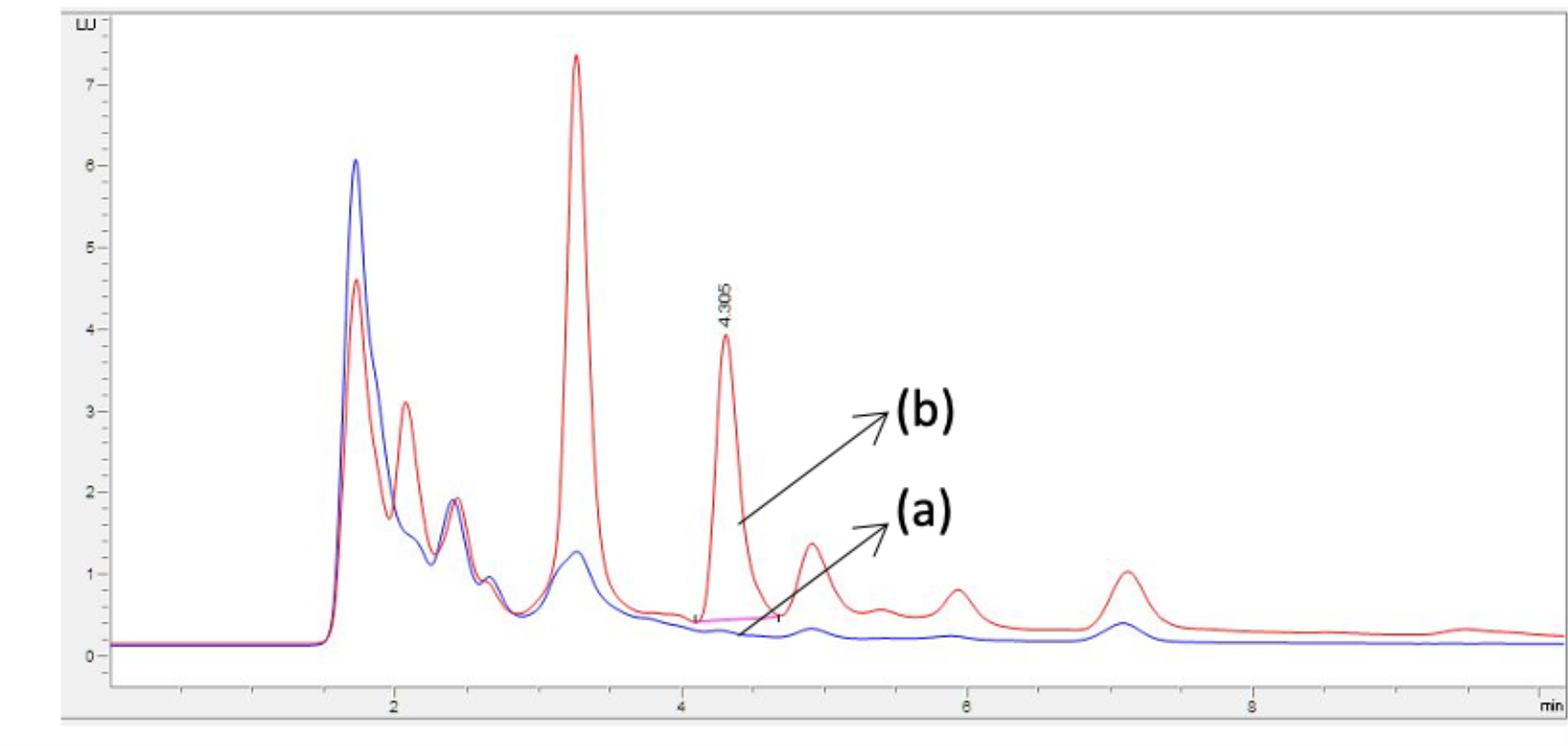Figure 3. An example of a recovery chromatogram of a grape juice (a) and a grape juice spiked with 2 μg/L of OTA (b).

Table 2. Characteristics of the method.

 Linearity range (μg/L) Determination coefficient (r2) LD (μg/L) LoQ (μg/L) Repeatability (n=7)   RSD% Reproducibility (n=7)   RSD% Recovery (%) 0.20 - 10 0.9999 0.12 0.22 4.79 5.17 104.2 ± 2.9

## 4.Bibliography

1. OIV. Compendium of International Methods of Analysis of Wines and Musts. Method OIV-MA-AS315-10.
2. EN ISO 3696. Water for analytical laboratory use — Specification and test methods (ISO 3696:1995).